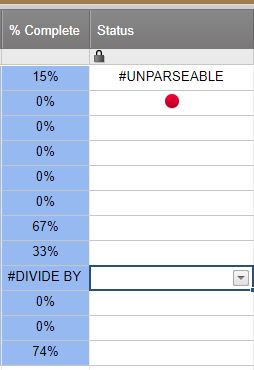#### Welcome to the Smartsheet Forum Archives

The posts in this forum are no longer monitored for accuracy and their content may no longer be current. If there's a discussion here that interests you and you'd like to find (or create) a more current version, please Visit the Current Forums.

# Automated RYG Balls

Hi everyone!

I am trying to automate some RYG balls.

Basically, i want to create a formula that says, if the number typed in one cell is between 0 and 5 make the ball in another cell go green.

If the number is between 5-10, make the ball go yellow etc.

Hope that makes sense. Thank you!!• 1. create a column with Type... "Symbols..." an selct the RYG you want

2. in the cells of this column type the following formula

=IF(AND(XY >= 0, XY < =5), "Green", IF(AND(XY>5,XY<=10),"Yellow",IF(XY>10,"Red","-")))

Where 'XY' is the reference of the cell where the data is, which you can lock on column or row by using "\$".

• Thanks guys! worked a treat!!• Hi,

Smartsheet novice here.

How can i create the following:-

IF status is not started - Set to Red

IF status is in progress - Set to Yellow

IF status is complete - Set to Green

Any help will be highly appreciated.

Thanks

VN

• VN,

The simplest version might look something like this:

=IF([% Complete]23 = 0, "Red", IF([% Complete]23 = 1, "Green", "Yellow"))

for row 23

Craig

• Hi everyone!

I am trying to automate some RYG balls.

probably more of the same, but I cannot make my formula work... what I want is to change the color according to percentages in a different column, 0%to 33% Red, 34%to66%Yellow and 67% to 100% turn to green.

I have used this formula, but is not working, any other thoughts around this?

=IF([% Complete]1<=33,"Red",IF(AND([% Complete]1>33,[% Complete]1<=66)=TRUE,"Yellow","Green"))

Thanks• Hi there, probably more of the same, but I cannot make my formula work... what I want is to change the color according to percentages in a different column, 0%to 33% Red, 34%to66%Yellow and 67% to 100% turn to green.

I have used this formula, but is not working, any other thoughts around this?

=IF([% Complete]1<=33,"Red",IF(AND([% Complete]1>33,[% Complete]1<=66)=TRUE,"Yellow","Green"))

I thought that you could know what is missing?

Regards• =IF([% Complete]1 <= 0.33, "Red", IF([% Complete]1 > 0.66, "Green", "Yellow"))

• Hello Can someone help me also, I have a date column and when the cells are empty I want the RYG Balls to have a RED color and Green when the cells have data (date). How do I do this.

• DLAGUS,

=IF(ISDATE([Date]23), "Green", "Red")

or

=IF(ISBLANK([Date]23), "Red", "Green")

for a column named [Date] and row 23.

I prefer the first. It will also be red for some other value (like a name or number)

I hope this helps.

Craig

• You are AMAZING!  Your formula finally helped me to automate my RYG balls column.  Is there an additional condition you can suggest to add to the end of the formula to leave the status ball cell blank if the cell where the date is located is blank?

Thanks so much for any suggestions!

Cecelia

• =IF(DY = "", "", IF(AND(XY >= 0, XY <= 5), "Green", IF(AND(XY > 5, XY <= 10), "Yellow", IF(XY > 10, "Red", "-"))))

✫✫✫ Where 'DY' is the reference of a cell (with a date for instance) to 'activate' the color balls: If DY is empty, then there will be no ball, otherwise the rule for coloring applies.

Where 'XY' is the reference of the cell where the data is, which you can lock on column or row by using "\$".

• Good day -- has anyone looked at a solution for a task list/project plan style sheet with sub-tasks to allow for the RYG value to "roll up" to determine the status of the section of tasks?

• What would be your algorithm then?

e.g. Do you want the parent to be red if any child is red?

or alt. Would you like to make a kind of an average value?

Pending you answer, consider the following formula that will address the number of red - yellow - green balls in children rows:

=COUNTIF(CHILDREN(), "Red") + "-" + COUNTIF(CHILDREN(), "Yellow") + "-" + COUNTIF(CHILDREN(), "Green")

Then it will only be a matter of adapting your algorithm to the information picked up from beneath. Let us klnow

• Example of formula to "average" answers.

=IF(SUM(COUNTIF(CHILDREN(), "Red") * (-1), COUNTIF(CHILDREN(), "Yellow") * 0, COUNTIF(CHILDREN(), "Green")) / COUNT(CHILDREN()) < -0.5, "Red", IF(SUM(COUNTIF(CHILDREN(), "Red") * (-1), COUNTIF(CHILDREN(), "Yellow") * 0, COUNTIF(CHILDREN(), "Green")) / COUNT(CHILDREN()) > 0.5, "Green", "Yellow"))

To avoid the cumbersome repetition of the COUNT formula, you can locate this very figure to an adjacent cell and run the IF-tests to it.

You could be even more sophisticated by weighing the different rows to give more sense to the parent conclusion ! ...

This discussion has been closed.